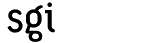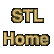# countCategory: algorithms Component type: function

### Prototype

Count is an overloaded name: there are two count functions.
```template <class InputIterator, class EqualityComparable>
iterator_traits<InputIterator>::difference_type
count(InputIterator first, InputIterator last,
const EqualityComparable& value);

template <class InputIterator, class EqualityComparable, class Size>
void count(InputIterator first, InputIterator last,
const EqualityComparable& value,
Size& n);
```

### Description

Count finds the number of elements in [first, last) that are equal to value. More precisely, the first version of count returns the number of iterators i in [first, last) such that *i == value. The second version of count adds to n the number of iterators i in [first, last) such that *i == value.

The second version of count was the one defined in the original STL, and the first version is the one defined in the draft C++ standard; the definition was changed because the older interface was clumsy and error-prone. The older interface required the use of a temporary variable, which had to be initialized to 0 before the call to count.

Both interfaces are currently supported , for reasons of backward compatibility, but eventually the older version will be removed.

### Definition

Defined in the standard header algorithm, and in the nonstandard backward-compatibility header algo.h.

### Requirements on types

For the first version, which takes three arguments:
• InputIterator is a model of Input Iterator.
• EqualityComparable is a model of Equality Comparable.
• InputIterator's value type is a model of Equality Comparable.
• An object of InputIterator's value type can be compared for equality with an object of type EqualityComparable.
For the second version, which takes four arguments:
• InputIterator is a model of Input Iterator.
• EqualityComparable is a model of Equality Comparable.
• Size is an integral type that can hold values of InputIterator's distance type.
• InputIterator's value type is a model of Equality Comparable.
• An object of InputIterator's value type can be compared for equality with an object of type EqualityComparable.

### Preconditions

• [first, last) is a valid range.
For the second version:
• [first, last) is a valid range.
• n plus the number of elements equal to value does not exceed the maximum value of type Size.

### Complexity

Linear. Exactly last - first comparisons.

### Example

```int main() {
int A[] = { 2, 0, 4, 6, 0, 3, 1, -7 };
const int N = sizeof(A) / sizeof(int);

cout << "Number of zeros: "
<< count(A, A + N, 0)
<< endl;
}
```

### Notes

 The new count interface uses the iterator_traits class, which relies on a C++ feature known as partial specialization. Many of today's compilers don't implement the complete standard; in particular, many compilers do not support partial specialization. If your compiler does not support partial specialization, then you will not be able to use the newer version of count, or any other STL components that involve iterator_traits.